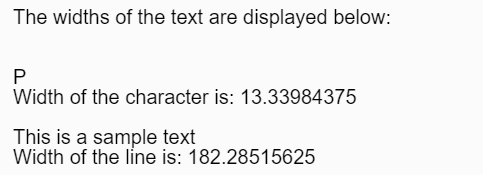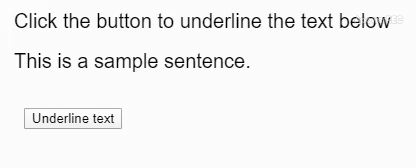# p5.js | textWidth() Function

The textWidth() function is used to calculate the width of the text given as parameter.

Syntax:

`textWidth( theText )`

Parameters: This function accepts a single parameter as mentioned above and described below:

• theText: It holds the string of which the width has to be measured.

Return Value: It returns a number which denotes the width of the given text.

Below examples illustrate the textWidth() function in p5.js:

Example 1:

 `let sampleChar = ``"P"``; ` `let sampleLine = ``"This is a sample text"``; ` `  `  `// Canvas area creating ` `function` `setup() { ` `  ``createCanvas(400, 200); ` `  ``textSize(20); ` `  ``text(``'The widths of the text are '` `        ``+ ``'displayed below:'``, 20, 20); ` `    `  `  ``// Checking textwidth sampleChar ` `  ``text(sampleChar, 20, 80); ` `  ``let charWidth = textWidth(sampleChar); ` `  ``text(``"Width of the character is: "`  `        ``+ charWidth, 20, 100); ` `    `  `  ``// Checking textwidth sampleLine  ` `  ``text(sampleLine, 20, 140); ` `  ``let lineWidth = textWidth(sampleLine); ` `  ``text(``"Width of the line is: "` `        ``+ lineWidth, 20, 160); ` `} `

Output:Example 2: Using text width messure to uderline the text.

 `let sampleText =  ` `    ``"This is a sample sentence."``; ` `  `  `// Canvas area creating ` `function` `setup() { ` `  ``createCanvas(400, 200); ` `  ``textSize(20); ` `  ``text(``'Click the button to underline'` `        ``+ ``' the text below'``, 20, 40); ` `  ``text(sampleText, 20, 80); ` `    `  `  ``// Creating button ` `  ``btn = createButton(``"Underline text"``); ` `  ``btn.position(30, 120); ` `  ``btn.mousePressed(underlineText); ` `} ` `  `  `// Messuring text width and ` `// creating underline ` `function` `underlineText() { ` `  ``let width = textWidth(sampleText); ` `  ``line(20, 90, width + 20, 90); ` `} `

Output:Online editor: https://editor.p5js.org/My Personal Notes arrow_drop_upCheck out this Author's contributed articles.

If you like GeeksforGeeks and would like to contribute, you can also write an article using contribute.geeksforgeeks.org or mail your article to contribute@geeksforgeeks.org. See your article appearing on the GeeksforGeeks main page and help other Geeks.

Please Improve this article if you find anything incorrect by clicking on the "Improve Article" button below.

Article Tags :

Be the First to upvote.

Please write to us at contribute@geeksforgeeks.org to report any issue with the above content.